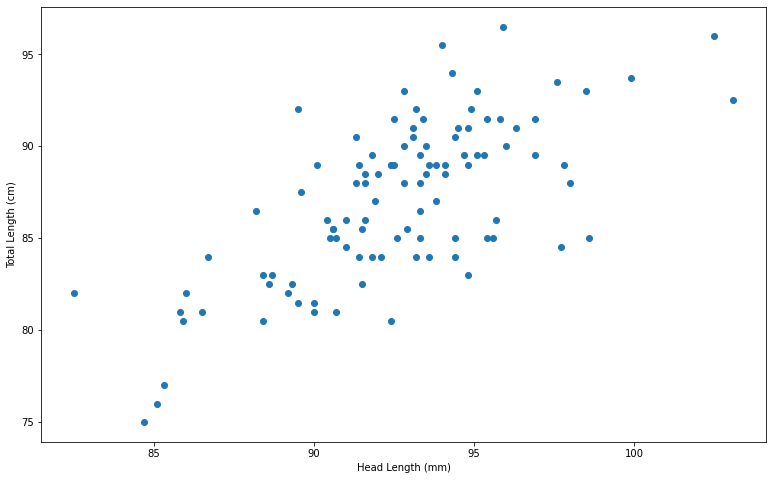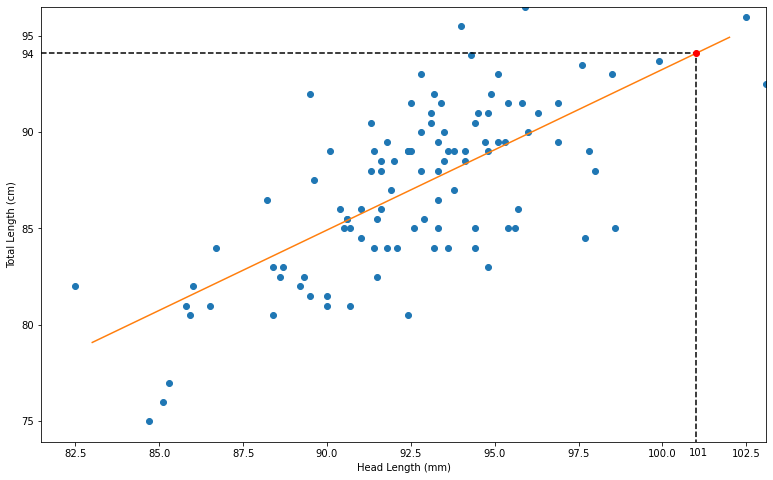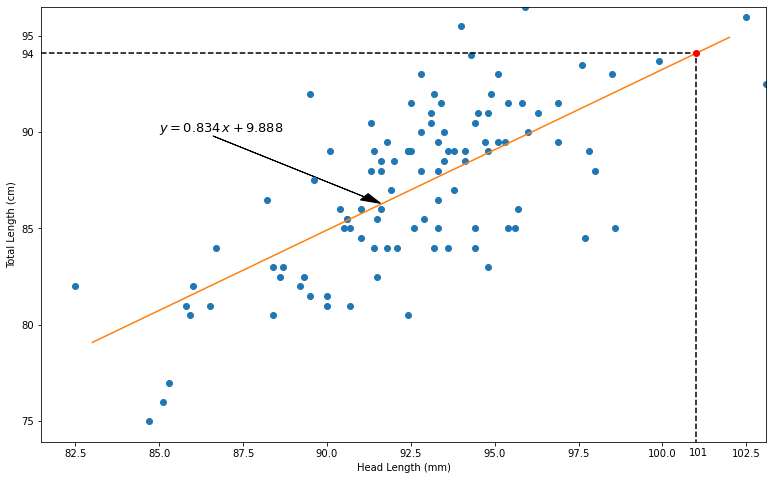# Linear Models and Regression¶

Sometimes, two data sets are related. Regression is a tool to help find and test the strength that relationship. In the simplest case, the relationship might be linear so we apply regression to a linear model.

This material is detailed in Chapter 8 of our text.

## Linear Models¶

One of the simplest types of relationships between two variables is a linear relationship - say, $$Y = aX+b.$$ In statistics, $X$ and $Y$ are typically random variables so we might ask questions like:

• Is there really a linear relationship between $X$ and $Y$?
• If so, what are the values of the parameters $a$ and $b$?
• What kind of predictions can we make using this relationship?
• How confident can be in those predictions?

### Relating head length and total length¶

Here's the plot of an example right from our text that relates the head length to total length for 104 possums.### The regression line¶

Here's the so-called "regression line" that models the data. We might infer from this line that a possum with a head length of 101 mm would have a total lenght of about 94 cm.## Lines in the plane¶

A line is the graph of an equation of the form $y=ax+b$.

The defining characteristic of such a graph is that it's slope is constant, i.e. if $y_1=ax_1+b$ and $y_2 = ax_2+b$, then

$$\frac{\Delta y}{\Delta x} = \frac{y_2-y_1}{x_2-x_1} = \frac{(ax_2+b)-(ax_1+b)}{x_2-x_1} = \frac{a(x_2-x_1)}{x_2-x_1} = a.$$

While that might seem complicated, it ultimately makes it easy to plot the line.

### Plotting a line¶

We can plot a line simply by plugging in a couple of points.

For example, to graph $y=2x+1$, plug in $x=0$ to get $y=0$ - that's one point!

Then plug in $x=1$ to get $y=3$ - that's another point!

Draw the line through both of those.

### The plot¶

Here's the plot of $y=2x+1$.

### An interactive plot¶

Here's a fun tool to see how the coefficients $a$ and $b$ affect the graph of $y=ax+b$.

### Using a regression line¶

In statistics, the forumula for a line will often be generated via software; you just need to interpret it. Thus it will be important for you to be able to plug a value like $x=1.234$ in to a line like $y=-5.8335x+0.8408$ to get a value. In this case: $$-5.8335\times1.234+0.8408 = −6.357739.$$

## Possums revisited¶

The possum example comes right from section 8.1 of our text. I've got the data stored on my website so we can read it in and run a regression analysis like so:

import pandas as pd
from scipy.stats import linregress

regression

LinregressResult(slope=0.8336697990278819, intercept=9.888233331751707, rvalue=0.6910936973935056, pvalue=4.680578654379419e-16, stderr=0.08632851506979797)

### The output¶

regression

LinregressResult(slope=0.8336697990278819, intercept=9.888233331751707, rvalue=0.6910936973935056, pvalue=4.680578654379419e-16, stderr=0.08632851506979797)

There are five features in this output. Of immediate importance are the slope and intercept, which describe the regression line. Those are basically the coefficients $a$ and $b$ we've been talking about. Thus, the line $$y = 0.8336697990278819x + 9.888233331751707$$ should be a reasonably good fit to the data. If we plug $x=101$ in, we should get about $y=94$ out:

0.8336697990278819*101 + 9.888233331751707

94.08888303356777

### Another look at the picture¶## Correlation¶

Let's take on more look at the output of that regression:

regression

LinregressResult(slope=0.8336697990278819, intercept=9.888233331751707, rvalue=0.6910936973935056, pvalue=4.680578654379419e-16, stderr=0.08632851506979797)

In addition to slope and intercept we see

• pvalue, which is a $p$-value for a hypothesis test that we'll talk about soon,
• stderr, which seems like it should be related to the $p$-value, and
• rvalue - ???

The "rvalue" is the the correlation between the two variables which is a measure of the strength of the linear relationship between them.

## Two more examples¶

Here are a couple more examples - one fun and one not.

### CFB¶

Correlating wins and losses to stats in College Football